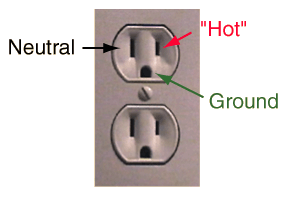# Household Use of Electric Energy

## Household circuits use energy, they do not use up electric charge.

The electric charge which flows into your house through your electric circuits carries with it useful energy which you can use for a variety of tasks. The US standard household circuit has an effective voltage of about 120 volts, and voltage represents energy per unit charge. 120 volts means 120 joules of energy transported by each coulomb of charge. A coulomb of charge is the amount of charge carried through a wire per second if an electric current of one ampere is flowing. So if you are operating an appliance with one ampere of current suppied at a voltage of 120 volts, you are using electric energy at the rate of 120 joules/second, or 120 watts of electric power.

When you turn on an electric appliance, you allow the 120 volts to force electric current through the appliance, analogous to using pressure from a pump to force water through a pipe. The energy which is carried by the charge is used by transforming it to accomplish heating, lighting, mechanical work (motors), etc. In the process, as the charge progresses through the appliance, its voltage drops from 120 volts to essentially zero volts with respect to the earth. This spent charge is then dumped to the earth through the ground wire. In the standard US receptacle, the charge at about zero volts is carried back to the electrical panel through the neutral wire and then transported to the earth by the ground wire attached to the electrical panel.

The use of electrical energy in a home is a part of a cycle which allows us to use the energy provided by a remote electric generator. We thereby take advantage of burning coal, oil, natural gas or using nuclear energy to provide the energy we need at home. At the generator, charge is taken from the earth at zero volts, and work is done on that charge to raise its voltage, providing a high energy AC voltage out of the generator. That voltage is raised even higher by transformers for efficient transport of the electric energy cross-country to your house. Other transformers lower the voltage to the standard supply voltages for your home. Then after using the energy on the charge, you return the spent charge back to the earth which acts as a large reservoir for electric charge.The high voltage (about 120 volts effective, 60 Hz AC) is supplied to the smaller prong of the standard polarized U.S. receptacle. It is commonly called the "hot wire". If an appliance is plugged into the receptacle, then electric current will flow through the appliance and then back to the wider prong, the neutral. The neutral wire carries the current back to the electrical panel and from there to the earth (ground). The ground wire is not a part of the electrical circuit, but is desirable for prevention of electric shock.

The two receptacles in a common "duplex" receptacle receive power from the same circuit leading from the main electrical supply panel. They are wired in parallel so that two appliances which are plugged into the receptacle receive the same voltage, but can draw different amounts of electric current. Parallel wiring is the standard for 120 volt circuits in the entire house, making possible the independent use of all appliances, supplied by the same voltage.

 Why is one prong wider? Example circuit
Index

Practical circuit concepts

 HyperPhysics*****Electricity and magnetism R Nave
Go Back